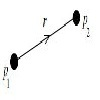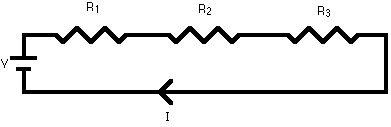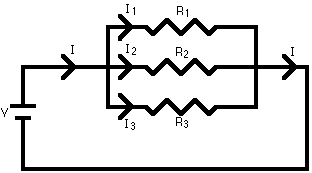#### You may also likeWhen resistances are connected in a line so that the current in the circuit has only one route to take the total resistance is the sum of the separate resistances. The resistances are said to be connected in series . In this diagram the total resistance $R$ is given by $R = R_1 + R_2 + R_3$.
 When resistances are connected in parallel so that, at a junction, the current flows along more than one route, the reciprocal of the total resistance is the sum of the reciprocals of the separate resistances. In this diagram the total resistance $R$ is given by $${1 \over R} = {1 \over R_1} + {1 \over R_2} + {1 \over R_3}.$$